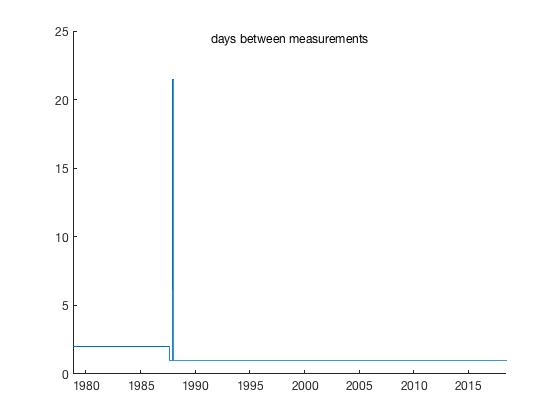# scatstat1 documentation

The scatstat1 function returns statistical values of all points within a given 1D radius of each value. This is similar to taking a moving mean, but points do not have to be equally spaced, nor do x values need to be monotonically increasing.

## Syntax

ybar = scatstat1(x,y,radius)
ybar = scatstat1(...,options)

## Description

ybar = scatstat1(x,y,radius) returns the mean of all y values within specified radius at each point x.

ybar = scatstat1(x,y,radius,fun) applies any function fun to y values, default fun is @mean, but could be @median, @nanstd, etc.

ybar = scatstat1(...,options) allows any options that the function accepts. For example, 'omitnan'.

## Example 1

Get the local median of all points within 10 units of each x point. Start by creating some random non-sorted data with N = 10,000 points:

N = 10000;
x = randi(300,N,1) + 20+3*randn(N,1) ;
y = 3*sind(x) + randn(size(x)) + 3;
plot(x,y,'k.')
axis tight
box offNow get the moving median of all points within 15 x units of each value:

yb = scatstat1(x,y,15,@median,'omitnan');

hold on
plot(x,yb,'bo')Note, this function does not interpolate or enforce any equal spacing. Final values correspond to each x point, which need not be equally spaced or sorted. Here's a zoom-in so you can see that the x values are not equally spaced:

axis([167 173 1 6])## Example 2: Sea ice data

From 1978 to 1988 the NSIDC sea ice time series contains about one measurement about every two days. After 1988, it's a daily time series. What if you want a 2 year moving average of sea ice time series, on this dataset which does not have constant temporal resolution?

Here's the situation:

load seaice_extent.mat % loads the sample data

figure
plot(t,extent_N)
axis tight
box off
ylabel 'northern hemisphere sea ice extent 1\times10^6 km)'Before 1988, the data is available every other day, and then there's a gap in the data, and then it's daily data. Plot the time between measurements (in days) as the gradient of the datenum values:

figure
ntitle 'days between measurements'
box offYou see, there's a gap.

For a two-year moving average (a radius of 1 year), convert the datetime format t to datenum format, and use scatstat1 with a radius of 365.25 days:

extent_N_2yr = scatstat1(datenum(t),extent_N,365.25);

figure(1) % goes back to the sea ice time series figure

hold on
plot(t,extent_N_2yr,'k','linewidth',2)And of course, you probably don't want to trust anything within 1 radius (1 year for the 2 year moving average) of the ends, which is why the strange wiggles appear on at the start and end of the time series.

## Author info:

This function was written by Chad A. Greene of the University of Texas at Austin's Institute for Geophysics (UTIG), June 2016.